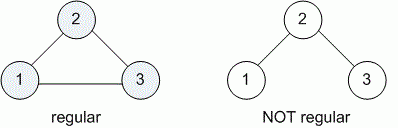favorite We need a little bit of your help to keep things running, click on this banner to learn more
Competitions

# Regular graph

Undirected graph is called regular, if all its vertices have the same degree.

Graph is given by list of edges. Check, is it regular.

#### Input

First line contains number n (1n100) of vertices and number m (mn (n - 1) / 2) of edges in a graph. Then given m pairs of numbers - the edges of graph.

#### Output

Print YES if graph is regular and NO otherwise.Time limit 1 second
Memory limit 128 MiB
Input example #1
```3 3
1 2
1 3
2 3
```
Output example #1
```YES
```
Input example #2
```3 2
1 2
2 3
```
Output example #2
```NO
```